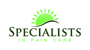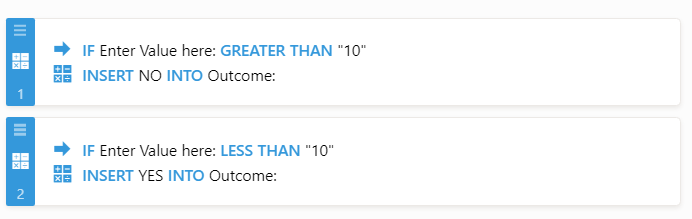# Question regarding conditional logic

•SPC11300
Asked on July 20, 2019 at 06:39 PM

I am putting together a form that asks certain questions and each question in the form has been assigned a risk value.  For example, a question might be something like: do you exercise?  They get 1 point for "no" and 2 points for "yes".

I am able to use the conditional logic feature to generate a score at the end of all the questions, but I would like to take that score and generate a risk level in a text format.  For example, if after all the questions the score is 15, I want to do something like "if risk score >= 15, then put 'high risk' in this box" or "if risk score < 15, the put 'low risk' in this box"

I cannot see how to do this after reading all of the documentation.

Here is my form, but it is hardly complete....I am just trying to conceptualize what I want the end product to be and I cannot generate a "risk score" based upon a certain risk number

https://hipaa.jotform.com/91998995132173

thanks

•SPC11300
Answered on July 20, 2019 at 09:35 PM

just to clarify a little more....

it would be nice if the conditional logic can use operators like less than and greater than a certain value

is that possible?

thanks

•jherwin
Answered on July 21, 2019 at 12:28 AM

You can assign calculation values ​​to each answer/option in your question. Then, use the form calculation widget to get the total or the value of all selected options/answers.

Guide:

And to show the "risk message" you need to use conditional logic for that.

•SPC11300
Answered on July 21, 2019 at 12:57 AM

You did not answer my question......

I have read all of those....

LET ME TYPE THIS IN CAPS AND WITH BOLD LETTERS: CAN YOU DO THE FUNCTIONS "LESS THAN" OR "GREATER THAN"?  For example, if value in field is < 10, put the word "YES" in this box; if value > 10, put the word "NO" in this box.

I did not see anything > or <

Do you have that function?  Where are the less than and greater than functions?

•jherwin
Answered on July 21, 2019 at 08:07 AM

Sorry for the confusion. Please use the "form calculation widget" as your textbox/text area so you can use greater than or less than functionality.

You can check my demo form here: https://form.jotform.com/92013619658966

The condition I used:Guide: How-to-Insert-Text-or-Calculation-into-a-Field-Using-Conditional-Logic

•SPC11300
Answered on July 21, 2019 at 02:12 PM

thank you....I got things working really well

clever stuff!

gml